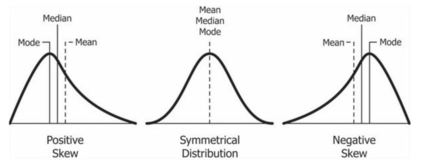## Skewness

Sample skewness is the measure of the asymmetry of the probability distribution of the data about its mean. It allows us to determine where most of the outliers are, which helps us determine which measure of central tendency is the most suitable for any given distribution.

It can be positive, zero or negative. For a unimodal distribution (a distribution with a single peak), negative skew commonly indicates that the tail is on the left of the distribution, with the median and mode to the right of the mean, while positive skew commonly indicates that the tail is on the right, with the median and mode to the left of the mean. Zero skew indicates that the tails on both sides of the mean balance out, as in the case for a symmetric distribution. However, this can also be true for an asymmetric distribution where one tail is long and thin, and the other is short but fat.Sample skewness, also known as the the Fisher-Pearson coefficient of skewness, is defined as$g_{1}=\frac{\frac{1}{n}\sum_{i=1}^{n}{(x_{i}-\bar{x})^{3}}}{(\frac{1}{n-1}\sum_{i=1}^{n}{(x_{i}-\bar{x})^{2}})^\frac{3}{2}}=\frac{\frac{1}{n}\sum_{i=1}^{n}{(x_{i}-\bar{x})^{3}}}{s^{3}}$

where s is the biased sample deviation$s=\sqrt{\frac{\sum_{i=1}^{n}(x_{i}-\bar{x})^{2}}{n}}$

The adjusted Fisher-Pearson coefficient of skewness is defined as$G_{1}=\frac{\sqrt{n(n-1)}}{n-2}g_{1}$

This provides a correction factor to adjust for sample size. This correction factor approaches 1 as$n$ gets large.

For the sample$X=\{1,1,2,1,2,3,2,1,0\}$, the sample mean$\bar{x}=1.4444$, the sample skewness is$g_{1}=\frac{\frac{1}{9}\sum_{i=1}^{9}{(x_{i}-1.4444)^{3}}}{(\frac{1}{8}\sum_{i=1}^{9}{(x_{i}-1.4444)^{2}})^\frac{3}{2}}=0.147986$

Translating this to code in NM Dev, we can use the class Skewness to compute the sample skewness using the above formula.

				
// create an array of doubles for our dataset
val values = doubleArrayOf(1.0, 1.0, 2.0, 1.0, 2.0, 3.0, 2.0, 1.0, 0.0)

// create the Skewness object
val skewness = Skewness(values)

println("Sample skewness: " + skewness.value())


				
Sample skewness: 0.1479860899612849